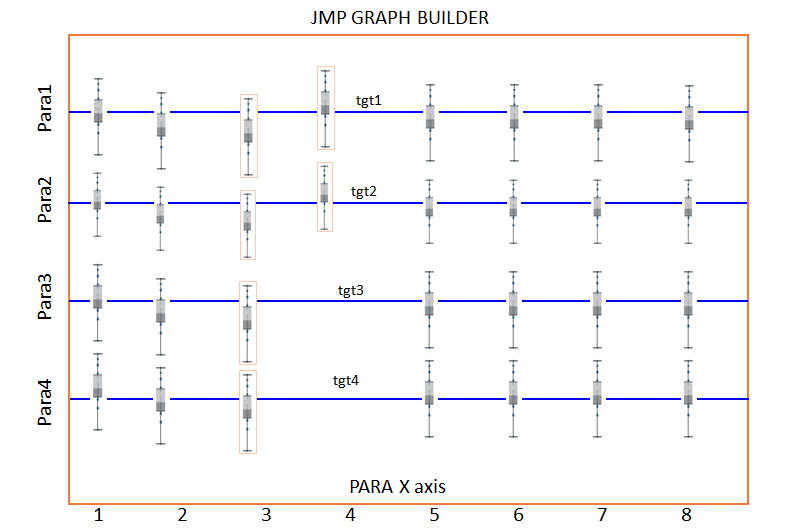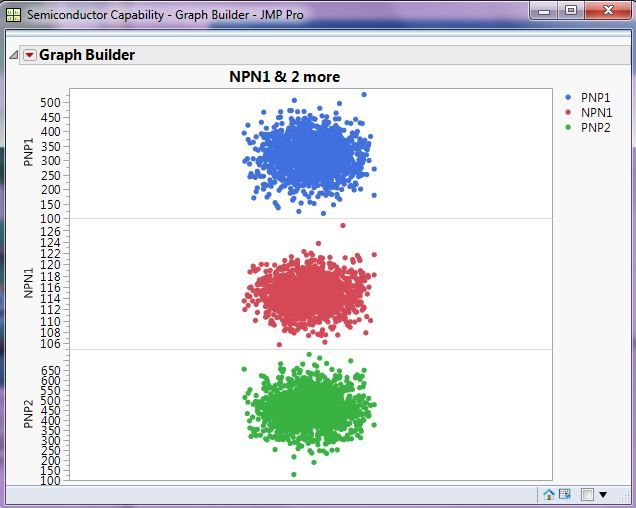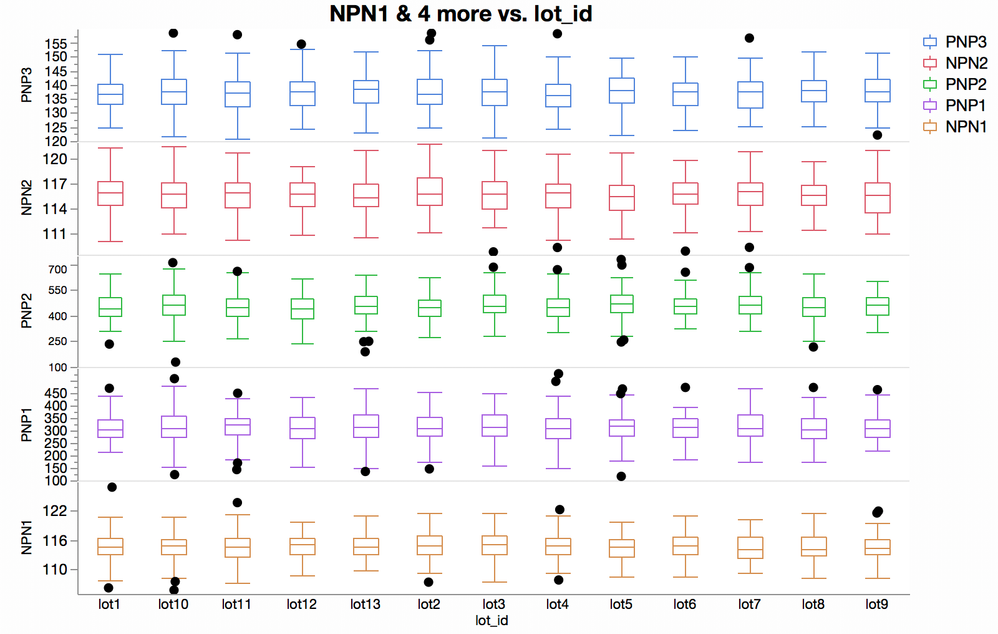Choose Language Hide Translation Bar
Highlighted

## Stacked parameter plot in graph builder

Hi All,

i am Trying to make a plot like the one shown in below cartoon in JMP.  can have multiple parameter on y axis as shown. These y parameter are of different scale. one parameter may be in 1e-9 and other may be in 1e+12. so need to have different y scale for different y parameters. after that each y parameter have their targets as hown in blue line. X axis is can be 1 parameter.

Is it somethign available in graph builder. or can be done using script?

Ram2 ACCEPTED SOLUTIONS

Accepted Solutions
Highlighted

## Re: Stacked parameter plot in graph builder

@ram

The documentation on how to do this is covered in:

Help==>Books==>Essential Graphing==>Create Separate Axes in the X and Y Zones

Multiple Y axes can easily be handled in Graph Builder``````Names Default To Here( 1 );
Open( "\$SAMPLE_DATA\Semiconductor Capability.jmp" );
Graph Builder(
Variables( Y( :PNP1 ), Y( :NPN1 ), Y( :PNP2 ) ),
Elements( Position( 1, 1 ), Points( Y, Legend( 5 ) ) ),
Elements( Position( 1, 2 ), Points( Y, Legend( 6 ) ) ),
Elements( Position( 1, 3 ), Points( Y, Legend( 7 ) ) )
);``````
Jim
Highlighted

## Re: Stacked parameter plot in graph builder

Like Jim said, but with an x variable and box plots:Each Y axis can have its own reference lines.

4 REPLIES 4
Highlighted

## Re: Stacked parameter plot in graph builder

@ram

The documentation on how to do this is covered in:

Help==>Books==>Essential Graphing==>Create Separate Axes in the X and Y Zones

Multiple Y axes can easily be handled in Graph Builder``````Names Default To Here( 1 );
Open( "\$SAMPLE_DATA\Semiconductor Capability.jmp" );
Graph Builder(
Variables( Y( :PNP1 ), Y( :NPN1 ), Y( :PNP2 ) ),
Elements( Position( 1, 1 ), Points( Y, Legend( 5 ) ) ),
Elements( Position( 1, 2 ), Points( Y, Legend( 6 ) ) ),
Elements( Position( 1, 3 ), Points( Y, Legend( 7 ) ) )
);``````
Jim
Highlighted

Thank you Jim.

Ram

Highlighted

## Re: Stacked parameter plot in graph builder

Like Jim said, but with an x variable and box plots:Each Y axis can have its own reference lines.

Highlighted

## Re: Stacked parameter plot in graph builder

Thank you Gregg

Article Labels

There are no labels assigned to this post.